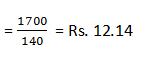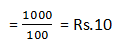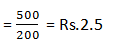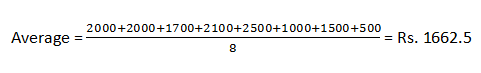New Students Offer - Use Code HELLO

# SBI PO Data Interpretation Series - Set 7

Directions (1-6): Study the following line graph carefully and answer the questions given below:

Assuming that there is no fixed component and all the units produced are sold in the same year.#### Ques 1.

In which of the following years is per unit cost the maximum?
1. 2009
2. 2010
3. 2007
4. 2011
5. 2013
4;
Suppose x units are produced in each year
In year 2007:
25 x = 4500
or, x = 180
profit = Rs. 2500
∴ CP = Rs. (4500-2500) = Rs. 2000
∴ Cost per unitIn year 2008:
20x = 4000
or, x = 200
∵ profit = Rs. 2000
∴ CP = Rs. (4000-2000) = Rs. 2000
∴ Cost per unitIn year 2009:
30x = 4200
or, x = 140
∵ profit = Rs. 2500
∴ CP = Rs. (4200-2500) = 1700
∴ Cost per unitIn year 2010:
30x = 5100
or, or x = 170
∵ profit = Rs. 3000
∴ CP = Rs. (5100 - 3000) = Rs. 12.35

In year 2011:
25x = 4000
or, x = 160
∵ Profit = Rs. 1500
∴ CP = Rs. (4000-1500) = 2500
∴ Cost per unitIn year 2012:
35x = 3500
or, x = 100
∵ profit = 2500
∴ CP = Rs. (3500 - 2500) = Rs. 1000
∴ Cost per unitIn year 2013:
25x = 3500
or, x = 140
∵ profit = 2000
∴ CP = Rs. (3500-2000)= Rs. 1500
∴ Cost per unitIn year 2014:
20x = 4000
or, x = 200
∵ profit = Rs. 3500
∴ CP = Rs. (4000 - 3500) = Rs. 500
∴ Cost per unitHence, in 2011 cost price per unit is the maximum.

#### Ques 2.

What is the average cost during the period 2007 to 2014?
1. Rs. 1600.5
2. Rs. 1862.5
3. Rs. 1962.5
4. Rs. 1752.5
5. Rs. 1662.5
5;
Cost = Revenue - Profit
Cost in 2007 = 4500 - 2500 = 2000
2008 = 4000 - 2000 = 2000
2009 = 4200 - 2500 = 1700
2010 = 5100 - 3000 = 2100
2011 = 4000 - 1500 = 2500
2012 = 3500 - 2500 = 1000
2013 = 3500 - 2000 = 1500
2014 = 4000 - 3500 = 500#### Ques 3.

If the SP per unit decreases by 20% during 2007 to 2010 and the cost per unit increases by 20% during 2011 to 2014. then during how many years is there no profit or loss?
1. None
2. One
3. Two
4. Four
5. Three

Ans : 1;
Year
Revenue
Total cost (old revenue – profit)
2007
80% of 4500 = 3600
4500-2500=2000
2008
80% of 4000 = 3200
4000-2000=2000
2009
80% of 4200 = 3360
4200-2500=1700
2010
80% of 5100 = 4080
5100-3000=2100
2011
4000
120% of (4000-1500 = 2500) = 3000
2012
3500
120% of (3500-2500=1000) = 1200
2013
3500
120% of (2500-1000=15000)=1800
2014
4000
120% of (4000-3500=500)=600

#### Ques 4.

What is the average of quantities sold during the period 2008 to 2012?
1. 146
2. 144
3. 154
4. 150
5. 158
3;
Average of quantities sold#### Ques 5.

if the SP per unit decreased by 25% during 2007 to 2010 and the CP per unit increased by 25% during 2011 to 2014 then the cumulative profit for the entire period 2007 to 2014 decreased by:
1. Rs. 5725
2. Rs. 5125
3. Rs. 5225
4. Rs. 5600
5. Rs. 5825
5;
Total decrease in revenue
= 25% of (4500 + 4000 + 4200 + 5100) = 4450
Total increase in cost
= 25% of (2500 + 1000 + 1500 + 500) = 1375
∴ Decrease in cumulative profit
= Total decrease in recenue + Total increase in cost
= 4450 + 1375 = Rs. 5825